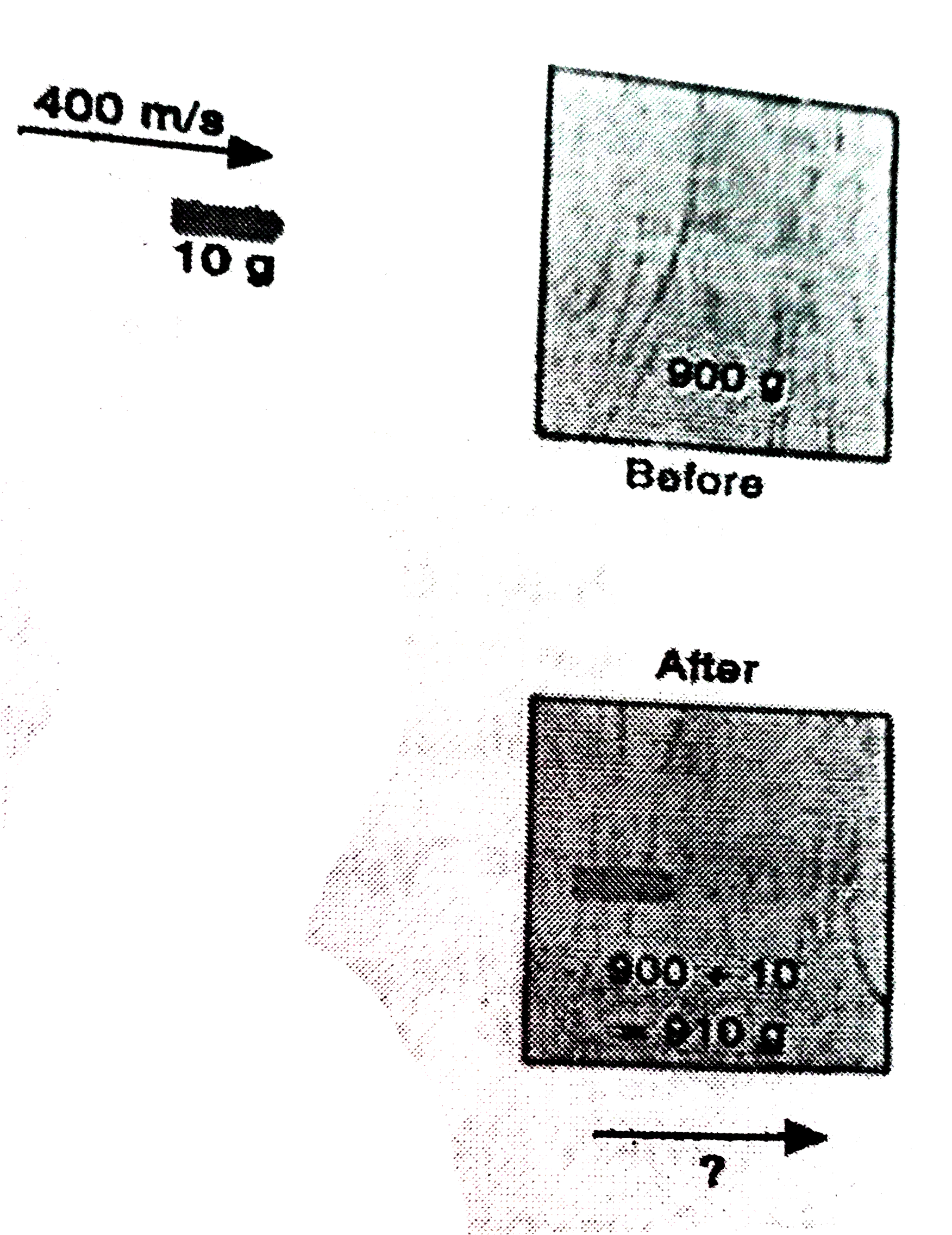Question

Updated on:30/05/2023

# A bullet of mass 10 g moving with a velocity of 400 m/s gets embedded in a freely suspended wooden block of mass 900 g. What is the velocity acquired by the block ?Text Solution

A
44 m/s
B
4.4 m/s
C
0.44 m/s
D
440 m/s
Solution
Here, Mass of the bullet,m1=10g
=101000kg
=0.01 kg
And, Velocity of the bullet, v1=400 m/s
So, Momentum of bullet=m1×v1
=0.01×400kg.m/s…….(1)
Now, this bullet of mass 10 g gets embedded into a wooden block of mass 900 g . So ,the mass of wooden blockk alongwith the embedded bullet will become 900+10=910 g. Thus,
Mass of wooden block+Bullet, m2=900+10
=910 g
=9101000 kg
=0.91 kg
And, Velocity of wooden block+bullet,v2=? (To be calculated)
So, Momentum of wooden block+bullet=m2×v2
=0.91×v2kg.m/s...(2)
Now, according to the law of conservation of momentum, the two momenta as given by equations (1) and (2) should be equal.
So, m1×v1=m2×v2
or 0.01×400=0.91×v2
And, v2=0.01×4000.91
=4.4 m/s
Thus, the velocity acquired by the wooden block (having the bullet embedded in it) is 4.4 metres per second.